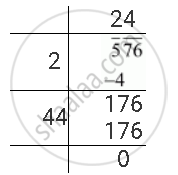Share

# Find the Square Root of the Following Number by Division Method. 576 - CBSE Class 8 - Mathematics

ConceptSquare Roots - Finding Square Root by Division Method

#### Question

Find the square root of the following number by division method.

576

#### Solution

The square root of 576 can be calculated as follows.∴ sqrt(576) = 24

Is there an error in this question or solution?

#### APPEARS IN

NCERT Solution for Mathematics Textbook for Class 8 (2018 to Current)
Chapter 6: Squares and Square Roots
Ex. 6.40 | Q: 1.09 | Page no. 107
Solution Find the Square Root of the Following Number by Division Method. 576 Concept: Square Roots - Finding Square Root by Division Method.
S